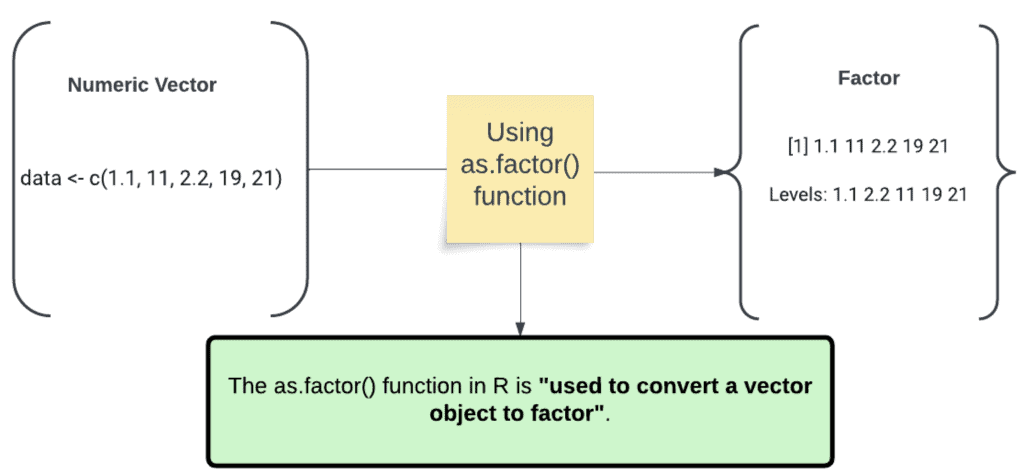# How to Use the as.factor() Function in RProcess diagram of as.factor() method

The as.factor() function in R is “used to convert a vector object to factor”. It takes a vector as a mandatory argument and returns a factor object.

## Syntax

``as.factor(input)``

## Parameters

input: The as.factor() function takes x as a column in an object of class or data frame.

## Return value

The as.factor() function returns a “factor object”.

## Example 1: Converting numeric vector to factor

``````data <- c(1.1, 11, 2.2, 19, 21)

as.factor(data)``````

Output

`````` 1.1 11 2.2 19 21
Levels: 1.1 2.2 11 19 21
``````

## Example 2: Converting character vector to factor

``````data <- c("zack", "synder", "cut")

as.factor(data)``````

Output

`````` zack synder cut
Levels: cut synder zack``````

## Example 3: Converting data frame column to factor

You can use the as.factor() function to convert a specific data frame column to a factor.

``````df <- data.frame(Singer = c("MJ", "Justin", "Drake", "Selena", "Rema", "Ed"),
Age = c(64, 30, 40, 30, 25, 38))

df\$Singer <- as.factor(df\$Singer)

print(df\$Singer)``````

Output

`````` MJ Justin Drake Selena Rema Ed
Levels: Drake Ed Justin MJ Rema Selena``````

## Difference between as.factor() and factor() in R

The main difference between as.factor() and factor() in R is that as.factor() is an abbreviated form of factor() that can sometimes run faster. The as.factor() coerces its argument to a factor, while factor() allows for more optional arguments.

Based on my experience, I created a table that summarizes the key differences between the two functions:

Function Description
as.factor() Converts its argument to a factor.
factor() Converts its argument to a factor and allows for more optional arguments, such as` levels`, `ordered`, and `exclude`.

That’s it.